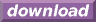#Calcute: freeware calculator

## Arctangent (arc-tangent, inverse tangent, atan)

The arc-tangent is the inverse of the tangent function. Calcute implements it with the atan function. The inverse tangent of a value x is the value y for which the tangent of y is x. In other words if y = atan(x) then x = tan(y). And since the tangent takes an angle as input parameter, the arctangent produces an angle as its output.

```atan(1)
0.785398163397
```

The atan value is expressed by Calcute using the currently-selected angle unit: radian, degree or gradient. In the above example, the result is in radians.

Note that the tangent function is periodic: its values repeat infinitely for angles that represent more than half of one complete rotation. Conversely, the arctangent is a multi-valued function: any parameter value matches an infinite number of possible angles. But the atan function generates a single value refered to as the principal value. This is the "nearest" valid angle that remains within half of one revolution. All other possibilities can be obtained by adding or subtracting any whole number of half rotations from the return value of atan.

In some mathematical texts and hand-held calculators, the notation tan-1 is sometimes used to represent the arc-tangent function. This is an unfortunate notational choice since it could also stand for the multiplicative inverse 1/tan, which is a different function.

Yet another term also used to represent the inverse tangent function is "arctan".